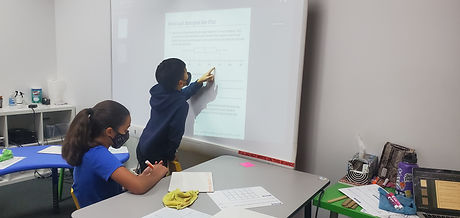## Mr. Jake

### Target 1​

###### Lesson Type:

New

Algebra

:

Variable

Solve mathematical problems by creating algebraic expressions.

###### 1:

Create equations using a variable to explain real-world situations.

###### 2:

Examine real-world problems and translate those patterns into algebraic expressions.

###### 3:

Use variables to represent numbers and write expression when solving real-world mathematical problems.

###### 4:

Use variable expressions to represent quantities that change in relationship to one another.

###### 5:

Reason with quantities by constructing algebraic expressions.

6th

###### Vocabulary:

Variable

Activities:

Solved a word problem as a class that involved two unknown values

Expressed one variable as an expression using the other given variable### Home Exploration

###### Guiding Questions:## Absent Students:

Palash, Amelia

### Target 2

:

###### 1:

Construct and read box and whisker plots.

###### 2:

Identify the parts of a box and whisker plot (median, quartile, upper quartile, lower quartile, and extremes).

###### 3:

Define median (the middle of a set of data), quartile (dividing data into four sections), upper quartile (the median of a quartile on the higher end of the range), lower quartile (the median of a quartile on the lower range), and extremes (the highest and lowest scores in the data range).

###### 4:

Draw a box and whisker plot to represent given data.

###### 5:

Divide data into quartiles (four sections).

7th

###### Vocabulary:

Quartile, Range, Median

Activities:

Created Box and Whisker plots from given data sets### Home Exploration

###### Guiding Questions:### Target 3

:

###### Vocabulary:

Activities:### Home Exploration